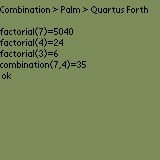Home > Palm > Samples > Combination > Forth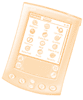Palm sample in Forth

####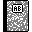Purpose

This is the Forth version of our combination application. It's one of the Palm Samples.

####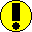Requirements

You need Quartus Forth (see tools section).

####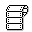Source code

Below is the .forth file.

```\ combination
\ Palm
\ Quartus Forth

: factorial ( n -- result )
dup                      \ Stack: n n
2 < if                   \ if n <= 1
drop 1 exit            \ return 1
else dup 1- recurse *  \ recurse
then
;

: combination ( n p -- result )
2dup           \ Stack: n p n p
- factorial    \ n p (n-p)!
rot factorial  \ p (n-p)! n!
rot factorial  \ (n-p)! n! p!
rot            \ n! p! (n-p)!
*              \ n! (p!*(n-p)!)
/              \ (n / (p!*(n-p)!))
;

\ Main entry point:
: go ( -- )
cr
." Combination > Palm > Quartus Forth" cr
cr
." factorial(7)=" 7 factorial . cr
." factorial(4)=" 4 factorial . cr
." factorial(3)=" 3 factorial . cr
." combination(7,4)=" 7 4 combination . cr
;
```

####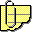Comments

Quartus Forth is a mobile tool. As such, it runs directly on your mobile device.

Copy and paste the source code above into a new memo with Palm Desktop. Run HotSync. The source code will be stored in a \Combination memo. Launch Quartus Forth, tap include combination to load the memo. To run the sample, enter go.

The core functions are : factorial, combination and go.

See for yourself how the RPN (Reverse Polish Notation) is amazing and unusual: 7 4 + (post-fixed notation) means 7 + 4 (unfixed notation).

After some practice, it becomes as legible - if not more - than our "traditional" unfixed notation.